# A guide to LLM inference and performance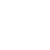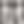Varun Shenoy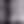Philip Kiely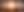### TL;DR

We want to use the full power of our GPU during LLM inference. To do that, we need to know if our inference is compute bound or memory bound so that we can make optimizations in the right area. Calculating the operations per byte possible on a given GPU and comparing it to the arithmetic intensity of our model’s attention layers reveals where the bottleneck is: compute or memory. We can use this information to pick the appropriate GPU for model inference and, if our use case allows, use techniques like batching to better utilize our GPU resources.

Many layers of abstraction sit between an ML model API and a bare-metal GPU. Developing strong mental models for these abstractions helps you control costs and improve performance during inference so that you get the most bang for your buck by fully exploiting the potential of your GPUs.

This guide will help you understand the math behind profiling transformer inference. As a concrete example, we’ll look at running Llama 2 on an A10 GPU throughout the guide. We’ll cover:

• Calculating the operations-to-byte (ops:byte) ratio of your GPU

• Calculating the arithmetic intensity of your LLM

• Comparing ops:byte to arithmetic intensity to discover if inference is compute bound or memory bound

• Practical strategies for optimizing memory-bound and compute-bound inference

Plus, we’ll check our work with real-world benchmarks of the model. You’ll come away from this guide with an understanding of the main bottlenecks for model serving and how to mitigate them.

One common workload is running a seven billion parameter LLM like Llama 2 or Mistral. Serving and LLM in production requires a GPU, but which one should we pick?

Suppose we pick an A10, a good middle ground between the lean T4 and the powerful (but pricey) A100. Here are the A10’s key specs.

### A10 key specs

Column AColumn B
FP3231.2 TF
TF32 Tensor Core62.5 TF | 125 TF*
BFLOAT16 Tensor Core125 TF | 250 TF*
FP16 Tensor Core125 TF | 250 TF*
INT8 Tensor Core250 TOPS | 500 TOPS*
INT4 Tensor Core500 TOPS | 1000 TOPS*
GPU Memory24 GB GDDR6
GPU Memory Bandwidth600 GB/s
Max TDP Power150W

There are three numbers we care about here when it comes to inference:

• FP16 Tensor Core: This is our compute bandwidth. We have 125 TFLOPS (teraflops, or a trillion float point operations per second) of available compute for models in half-precision (also known as FP16). Half-precision is a binary number format that occupies 16 bits per number, as opposed to full-precision, which refers to a binary format that utilizes 32 bits per number. For many ML applications, using half-precision is a practical choice as it requires less memory without losing accuracy. In this blog post, we ignore datasheet values associated with sparsity (denoted by an asterisk).

• GPU Memory: We can quickly estimate the size of a model in gigabytes by multiplying the number of parameters (in billions) by 2. This approach is based on a simple formula: with each parameter using 16 bits (or 2 bytes) of memory in half-precision, the memory usage in GB is approximately twice the number of parameters. Therefore, a 7B parameter model, for instance, will take up approximately 14 GB of memory. Why does this matter? Well, with our A10's 24 GB of VRAM, we can comfortably run a 7B parameter model and still have about 10 GB of memory remaining as a buffer. This spare memory plays an important role in model execution, something we will elaborate on later.

• GPU Memory Bandwidth: We can move 600 GB/s from GPU memory (also known as HBM or high bandwidth memory) to our on-chip processing units (also known as SRAM or shared memory).

## Calculating the operations per byte (ops:byte) ratio

Using these numbers, we can calculate the ops:byte ratio of our hardware. This tells us how many floating point operations per second (FLOPS) we can complete for every byte of memory we access.

Given the numbers from the spec sheet, we calculate the ops:byte ratio for the A10:

``````ops_to_byte_A10
= compute_bw / memory_bw
= 125 TF / 600 GB/S
= 208.3 ops / byte``````

This means to take full advantage of our compute resources, we have to complete 208.3 floating point operations for every byte of memory access.

• If we find ourselves only able to complete fewer than 208.3 operations per byte, our system performance is memory bound. This essentially means that the speed and efficiency of our system are constrained by the rate at which we can transfer data or the input-output operations that it can handle.

• If we want to do more than 208.3 floating point operations per byte, our system is instead compute bound. In this state, our effectiveness and performance are restrained not by the memory, but rather the number of compute units that our chip possesses.

It’s essential to know if we are compute bound or memory bound so we know where to focus optimization efforts.

## Calculating arithmetic intensity

To determine whether we’re memory bound or compute bound, we need to calculate the arithmetic intensity of our 7 billion parameter LLM, then compare it to the ops:byte ratio we just calculated for our GPU. Arithmetic intensity is the number of compute operations an algorithm takes divided by the number of byte accesses it requires and is a hardware-agnostic measurement.

The most computationally expensive parts of our 7B parameter LLM are the attention layers, which ensure next token predictions are weighted based on the relevance of previous tokens. Because attention layers are the most computationally demanding part of the inference, we’ll calculate our arithmetic intensity there.

Understanding attention layers requires getting just a bit more specific with how the model works under the hood. When sampling from a transformer, there are two phases:

1. Prefill: In the first phase, the model ingests your prompt tokens in parallel, populating the key-value (KV) cache. The KV cache can be thought of as the state for your model, nestled within the attention operation. During the prefill, no tokens are being generated.

2. Autoregressive sampling: In the second phase, we leverage our current state (stored in the KV cache) to sample and decode the next token. We pay a small price in storage in order to not recalculate the cache for every single new token. Without the KV cache, every successive token would take longer to sample because we would have to pass all previously seen tokens through the model.

### Breaking down the attention equation

Below is the equation for attention in LLM inference. We’ll go through this equation step by step to determine what requires memory movement and what requires compute operations, which we can then compare to find the arithmetic intensity that we’re looking for.The authors of the FlashAttention paper have a great implementation for the standard attention algorithm. This framing will make it easier for us to calculate memory and compute in the algorithm.Eagle-eyed readers might notice this algorithm drops the scaling by `sqrt(d_k)`. It’s a minor factor that we can safely ignore.

All three steps follow the same pattern: load values from memory, perform a computation, and store the results of that computation to memory. In the algorithm:

• `N` is the sequence length of the LLM, which sets the context window.

• For Llama 2 7B, `N = 4096`.

• `d` is the dimension of a single attention head.

• For Llama 2 7B, `d = 128`.

• `Q`, `K`, and `V` are all matrices used to compute attention.

• Their dimensions are `N` by `d`, or in our case `4096x128`.

• `S` and `P` are both matrices calculated during the equation.

• Their dimensions are `N` by `N`, or in our case `4096x4096`.

• `O` is the output matrix with the results of the attention calculation.

• `O` is an `N` by d matrix, or in our case `4096x128`.

• HBM is high bandwidth memory.

• From the data sheet, we know that we have 24 GB of HBM on the A10 operating at 600 GB/s.

With these figures in mind, let’s break down the standard attention algorithm for each line in the implementation, which we’ll then sum to find the total compute and memory costs of running the algorithm.

Line in algorithmLoad from memoryComputeStore to memory
Line 1:size_fp16 * (size_Q + size_K)cost_dot_product_QK * size_Ssize_fp16 * size_S
= 2 * 2 * (N * d)= (2 * d) * (N * N)= 2 * (N * N)
Line 2:size_fp16 * size_Scost_softmax * size_Psize_fp16 * size_P
= 2 * (N * N)= 3 * (N * N)= 2 * (N * N)
Line 3:size_fp16 * (size_P + size_V)cost_dot_product_PV * size_Osize_fp16 * size_O
= 2 * ((N*N) + (N * d))= (2 * N) * (N * d)= 2 * (N * d)

We calculate total memory movement by summing the first and third columns (the loads from and stores to memory).

``````total_memory_movement_in_bytes:
= (2 * 2 * (N * d)) + (2 * (N * N)) + (2 * ((N*N) + (N * d))) + (2 * (N * N)) + (2 * (N * N)) + (2 * (N * d))
= 8N^2 + 8Nd bytes``````

And calculate total compute by summing the second column (the compute on the loaded data).

``````total_compute_in_floating_point_ops:
= ((2 * d) * (N * N)) + (3 * (N * N)) + ((2 * N) * (N * d))
= 4(N^2)d + 3N^2 ops``````

The arithmetic intensity can be calculated as follows.

``````arithmetic_intensity_llama
~= total compute / total memory movement
= 4d(N^2) + 3N^2 ops / 8N^2 + 8Nd bytes
= 62 ops/byte for Llama 2 7B``````

## Discovering our inference bottleneck

Our arithmetic intensity for Llama 2 7B is 62 operations per byte, which is way less than our A10’s ops:byte ratio of 208.3.

Thus, during the autoregressive phase, our model is memory bound. In other words, in the time it takes us to move a single byte from memory to compute, we could have completed many, many more calculations than just on that byte.

This is a problem. We’re paying good money to keep our GPUs up, but are not using the compute that’s available to us.

## Batching memory-bound processes on a GPU

One solution is to leverage the extra on-chip memory to run forward passes through our model in batches. In other words, we can wait a couple hundred milliseconds to rack up a few requests and run them all in a single pass instead of greedily processing requests as they arrive. This enables us to reuse parts of the model that we’ve already loaded into the GPU’s SRAM.

Batching increases the model’s arithmetic intensity by doing more computation for the same number of loads and stores from memory, which in turn reduces the degree to which the model is memory bound.

How big can we make our batches? Recall that we have 10 GB of memory left on our A10 after loading in our 7B parameter model:

``24 GB - (2 * 7GB) = 10GB``

Now, the question is how many sequences can we fit in that spare GPU memory at once?

To calculate this figure, we’ll need to return to the KV cache. Recall that during the prefill step in the attention layer, we populate the KV cache based on the prompt, or input sequence.

The KV cache contains the matrices K and V that we used during attention calculation. We need some of the values from earlier and a couple of new ones to calculate the size of the KV cache:

• `d`, which can be notated as `d_head`, is the dimension of a single attention head.

• For Llama 2 7B, `d = 128`.

• `n_heads` is the number of attention heads.

• For Llama 2 7B, `n_heads = 32`.

• `n_layers` is the number of times the attention block shows up.

• For Llama 2 7B, `n_layers = 32`.

• `d_model` is the dimension of the model. `d_model = d_head * n_heads`.

• For Llama 2 7B, `d_model = 4096`.

It’s worth noting that d_model being the same as N (the context window length) is coincidental. As the Llama paper shows, other sizes of Llama 2 have a larger `d_model` (see the “dimension” column).At half precision (FP16), each floating point number takes 2 bytes to store. There are 2 matrices, and to calculate the KV cache size, we multiple both by `n_layers` and `d_model`, yielding the following equation:

``````kv_cache_size
= (2 * 2 * n_layers * d_model) bytes/token
= (4 * 32 * 4096) bytes/token
= 524288 bytes/token
~ 0.00052 GB/token``````

Given that the KV cache requires 524288 bytes per token, how large can the KV cache be in terms of tokens?

``````kv_cache_tokens
= 10 GB / 0.00052 GB/token
= 19,230 tokens``````

Our KV cache can comfortably accommodate 19,230 tokens. Thus, for Llama 2's standard sequence length of 4096 tokens, our system has the bandwidth to handle a batch of 4 sequences concurrently.

In summary, to make the most of the compute capacity that we’re paying for, we want to batch 4 requests at a time during inference to fill our KV cache. This will increase our throughput.

If you are using LLMs to process a large queue of documents asynchronously, batching is a great idea. You will process the queue much faster than if you were to process each element individually, and can schedule the inference calls so that they fill up batches quickly, minimizing the impact on latency.

## Evaluating GPUs for LLM inference

In some cases, batching may not make sense. For example, if you’re building a user-facing chatbot, your product is much more sensitive to latency, so you can’t wait for a batch to fill before running inference. What should we do in this case?

One option is to recognize that we won’t be able to fully utilize our GPU’s on-chip memory, and downsize. For example, we can move to a T4 GPU, which has 16 GB of VRAM. This can still hold our 7B parameter model, but there’s much less leftover capacity — only 2 GB — for batching and KV caching.

However, a T4 GPU is usually slower than an A10. And an A100, while more powerful, is also more expensive. We can quantify this difference by calculating some simple lower bounds on inference times.

### Generating a single token on each GPU

Recall that during the autoregressive part of generation, we are memory bandwidth bound if our batch size is 1. Let’s quickly calculate how long it takes to generate a single token using the following equation:

``time/token = total number of bytes moved (the model weights) / accelerator memory bandwidth``
• On an T4: `(2 * 7B) bytes / (300 GB/s)` = 46 ms/token

• On an A10: `(2 * 7B) bytes / (600 GB/s) `= 23 ms/token

• On an A100 SXM 80 GB: `(2 * 7B) bytes / (2039 GB/s)` = 6 ms/token

These estimates show that an A10 is twice as fast as a T4, and an A100 is nearly four times faster than an A10 (and thus eight times faster than a T4).

These numbers are only an approximation, because they assume there is zero communication within the GPU during inference, zero overhead on each forward pass, and perfect parallelization during computation.

### Prefilling with batched prompt tokens on each GPU

We can also compute the time it takes for the prefill section assuming that we batch all of the prompt tokens into a single forward pass. Let’s assume that the prompt has 350 tokens, for simplicity, and that the limiting bottleneck is compute, and not memory.

``Prefill time = number of tokens * ( number of parameters / accelerator compute bandwidth)``
• On a T4: `350 * (2 * 7B) FLOP / 65 TFLOP/s` = 75 ms

• On an A10: `350 * (2 * 7B) FLOP / 125 TFLOP/s` = 39 ms

• On an A100 SXM 80 GB: `350 * (2 * 7B) FLOP / 312 TFLOP/s` = 16 ms

### Estimating total generation time on each GPU

Assuming we allow for 150 completion tokens (and we suppress any stop tokens), our total generation time will be as follows.

``Total generation time = prefill time + number of tokens * time/token``
• On a T4 = `75 ms + 150 tokens * 46 ms/token` = 6.98 s

• On an A10 = `39 ms + 150 tokens * 23 ms/token` = 3.49 s

• On an A100 SXM 80 GB: `16 ms + 150  tokens * 6 ms/token` = 0.92s

Factoring in GPU prices, we can look at an approximate tradeoff between speed and cost for inference. The specifics will vary slightly depending on the number of tokens used in the calculation.

GPUInference timeList priceSpeedup vs T4Cost vs T4
T46.98 seconds\$0.01052/minn/an/a
A103.49 seconds\$0.02012/min~ 2x~ 2x
A1000.92 seconds\$0.10240/min~ 7.5x~ 10x

## Benchmarks for Llama 2 7B Chat on NVIDIA GPUs

In Physics class, many homework problems take place in a frictionless vacuum. The estimates we’ve been making have taken place in a similarly theoretical circumstance. Let’s compare them to real world figures.

The numbers we have calculated are underestimates of inference time because they don’t account for factors like GPU communication costs, network delays, and imperfect utilization. In the real world, these factors can make inference take up to twice as long.

The practical benchmark uses Llama 2 7B Chat on Baseten and measures the end-to-end latency on applicable GPUs without any optimizations apart from using ExLlama V2.

We use a standardized prompt that has an exact input length of 350 tokens according to the Llama 2 tokenizer. The prompt is shared below. The text to summarize is pulled from How to Do Great Work by Paul Graham.

``````Write a concise summary of the following:

"The first step is to decide what to work on…[excerpt continues long enough to make the prompt exactly 350 tokens]"

CONCISE SUMMARY:``````

We also allowed a maximum of 150 output tokens, with no stop token, which guaranteed that every query would spend 500 tokens in total. Therefore, we can use calculations from above to predict how long these queries will take.

The predictions are shown in dark green, while the measured generation times are shown in a lighter shade. The expected calculations are in the right ballpark, but always fall short because they make generous assumptions when it comes to the parallelization of work and ignore communication delays within the GPU.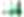Comparison of generation time and predicted time across instance types

## Optimizing LLM model inference with transformer math

We want to make the most of compute capacity during LLM inference, but we can’t do that when we’re memory bound. Calculating the operations per byte possible on a given GPU and comparing it to the arithmetic intensity of our model’s attention layers lets us understand if we’re memory bound or compute bound.

When memory bound, batching lets us make the most of our compute capacity, though batching isn’t possible for many latency-sensitive use cases. When we start with a strong latency requirement, we can use similar calculations to estimate which GPUs can meet our needs.

As useful as these theoretical calculations are, it’s alway essential to check them against real-world benchmarks to account for factors like communication costs and network delays.

Looking under the hood of LLM inference is fascinating, and there’s always further to dig. Here are some great resources for learning more: